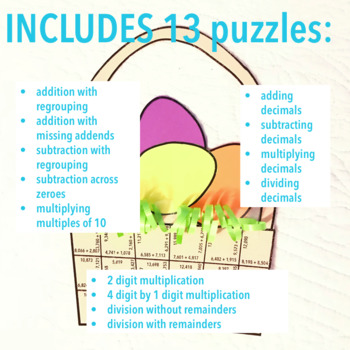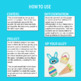Subject
Resource Type
Format
PDF (10 MB|16 pages)
Standards
\$5.00
• Product Description
• Standards

Students will be engaged in this EASTER math project by putting together tarsia puzzles to ultimately create AN EASTER BASKET! Includes 13 PUZZLES!

This resource may be used as centers or as an individual or group project. The only materials needed besides what's included are glue and paper colors of choice.

Skills Included:

Subtraction with Regrouping

Subtraction Across Zeroes

Multiplying Multiples of 10

2 digit by 2 digit multiplication

4 digit by 1 digit multiplication

Division with No Remainders

Division with Remainders

Subtracting Decimals

Multiplying Decimals

Dividing Decimals

Your students will be ready to assess across math skills and ready to celebrate after completing this engaging activity!

to see state-specific standards (only available in the US).
Add, subtract, multiply, and divide decimals to hundredths, using concrete models or drawings and strategies based on place value, properties of operations, and/or the relationship between addition and subtraction; relate the strategy to a written method and explain the reasoning used.
Find whole-number quotients of whole numbers with up to four-digit dividends and two-digit divisors, using strategies based on place value, the properties of operations, and/or the relationship between multiplication and division. Illustrate and explain the calculation by using equations, rectangular arrays, and/or area models.
Fluently multiply multi-digit whole numbers using the standard algorithm.
Find whole-number quotients and remainders with up to four-digit dividends and one-digit divisors, using strategies based on place value, the properties of operations, and/or the relationship between multiplication and division. Illustrate and explain the calculation by using equations, rectangular arrays, and/or area models.
Multiply a whole number of up to four digits by a one-digit whole number, and multiply two two-digit numbers, using strategies based on place value and the properties of operations. Illustrate and explain the calculation by using equations, rectangular arrays, and/or area models.
Total Pages
16 pages
N/A
Teaching Duration
Lifelong tool
Report this Resource to TpT
Reported resources will be reviewed by our team. Report this resource to let us know if this resource violates TpT’s content guidelines.
• Ratings & Reviews
• Q & A

Teachers Pay Teachers is an online marketplace where teachers buy and sell original educational materials.